### 11. The Sunyaev-Zel'dovich effect interpreted in cosmological terms

The simplest cosmological use of the Sunyaev-Zel'dovich effect is to prove that the CMBR is genuinely a cosmological phenomenon: the appearance of an effect from a cluster of galaxies at z = 0.5455 (CL 0016+16) proves that the CMBR originates at z > 0.54, higher-redshift detections push this limit even further. However, it is as a probe of cosmological parameters, and as a distance-independent probe of earlier phases of the Universe that the Sunyaev-Zel'dovich effect has attracted most interest, and such uses of the effect are the focus of this section.

### 11.1 Cosmological parameters

The basis of the use of the Sunyaev-Zel'dovich effect as a tracer of cosmological parameters was given in Sec. 4.1. The essence of the idea is the same as for other distance-measuring techniques that depend on a comparison of the emission and absorption of radiation from gas: the surface brightness of the gas in emission is proportional to the line-of-sight integral of some density squared,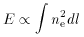(104)

while the absorption of some background source of radiation is proportional to the optical depth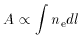(105)

Thus if both the emission from the gas, E, and its absorption, A, can be measured, the quantity A2 / E is a density-weighted measure of the path-length through the gas. If the structure of the gas is known, and its angular size,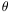, can be measured, then the angular diameter distance of the gas can be estimated from A2 / (E).

Although this technique may eventually be applied using only X-ray data (Krolik & Raymond 1988), it is currently used for the measurement of distances using a combination of X-ray and the Sunyaev-Zel'dovich effect data (Gunn 1978; Silk & White 1978; Birkinshaw 1979; Cavaliere et al. 1979). The emission of gas in a cluster of galaxies is measured by its X-ray surface brightness,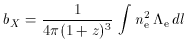(106)

wheree(E, Te) is the X-ray spectral emissivity of the cluster gas (Sec. 4.1), while the absorption by the gas is measured by the thermal Sunyaev-Zel'dovich effect, which can be expressed as an intensity change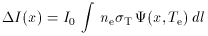(107)

at dimensionless frequency x = h/ kBTrad, where I0 = (2 h / c2) [(kBTrad / h)]3 is a scale intensity and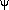(x,Te) is the dimensionless form of the frequency-dependent, relativistic, spectrum of the effect (from equation 51),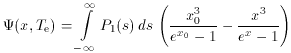(108)

with s = ln (x / x0) (see Secs 3.2 and 3.3; this form is used by Holzapfel et al. 1997a).

Since the technique compares the angular size of a cluster of galaxies with a measure of the line-of-sight size of the cluster, it is important to have a model for the structure of the gas so that the relationship between the projected quantities bX andI can be calculated. It is convenient to express the electron concentration and temperature in terms of reference values (chosen as the central values here, although the values at any fiducial point can be used) and dimensionless form factors describing the angular structure of the gas in density, fn(,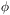,), and temperature, fT(,,). The angular variables are, the angle from the reference line of sight through the cluster center,= l / DA, an angular measure of distance down the line of sight, and, an azimuthal angle about the line of sight. DA is the angular diameter distance of the cluster. Then the electron density and temperature at some location, r, are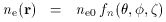(109)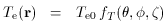(110)

and the energy loss and spectrum functions may be written in terms of similar form factors which depend on fn and fT in complicated ways,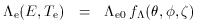(111)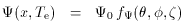(112)

(Birkinshaw et al. 1991; Holzapfel et al. 1997a). The X-ray surface brightness and the thermal Sunyaev-Zel'dovich effect intensity change can then be expressed in terms of physical constants and angular structure factors, as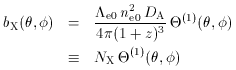(113)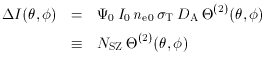(114)

with the structural information for the cluster contained in the angles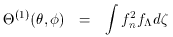(115)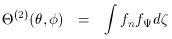(116)

which describe the shapes of the X-ray and Sunyaev-Zel'dovich effects that the model gas distribution would produce.

An absolute distance for a cluster is then found by fitting the X-ray and Sunyaev-Zel'dovich effect data to models of the form (113) and (114) to deduce NX and NSZ, and calculating the angular diameter distance using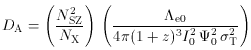(117)

(Holzapfel et al. 1997a), or equivalently from the form given by Birkinshaw et al. (1991) in their equation 39, if brightness temperature rather than intensity is used as the Sunyaev-Zel'dovich effect observable. The value of the Hubble constant is then obtained from the measured redshift of the cluster and the value of DA under some assumption about the value of q0 using equation (71).

This is a direct method of measuring the distance of a cluster of galaxies and the value of the Hubble constant: it can be applied at large cosmological distances without any intervening chain of distance estimators (as in the usual distance ladder). The distance estimate relies on simple physics - the properties of a fully-ionized gas held nearly in hydrostatic equilibrium in the gravitational potential well of a cluster of galaxies. The basis of this distance estimate can therefore be tested by making a detailed study of the properties of the cluster being used as a cosmological tracer and the population of similar clusters. It is also important that in this method each cluster of galaxies is treated as an individual - the evolutionary peculiarities of a distant cluster need not affect the distance estimate provided that the physical state of the intracluster gas is understood. Of course, if the cluster gas has much small-scale density and temperature structure, it may be difficult to obtain good models for the form factors (109) - (112), and there may be a substantial systematic error in the distance estimate. Some protection against this systematic error can be obtained by cross-checking the independent results that are obtained from a number of clusters.

The measurement of the values of NX and NSZ from the X-ray and Sunyaev-Zel'dovich effect data not only requires knowledge of the form factors fn, fT, f, and fbut also the fiducial electron temperature of the cluster, T0, since T0 is an implicit variable in (117), where it enters in bothe0 and0. Even with the help of the resolved X-ray spectroscopy that will become available on the next generation of X-ray telescopes (such as AXAF), it is not possible to use the X-ray or Sunyaev-Zel'dovich effect data to measure these three-dimensional form factors. Therefore, the calculation proceeds by adopting some parameterized models for the electron concentration and temperature as functions of position which are consistent with the X-ray image and spectroscopy and the Sunyaev-Zel'dovich effect data. The normalizations NX and NSZ that are found are then dependent on the unknown structural parameters of the model atmosphere after any adjustable parameters have been determined.

Rephaeli & Yankovitch (1997) have recently pointed out that for good accuracy in calculating cluster distances in this way, it is important to the full relativistic formalism (Gould 1980) to calculate the value ofe0 and ffor X-ray emission from the cluster gas, just as the relativistic expression for the Sunyaev-Zel'dovich effect (51) must be used. Hughes & Birkinshaw (1998) have shown that the size of the relativistic correction in Rephaeli & Yankovitch's work is excessive, apparently because of their use of an equation containing a typographical error in Gould (1980). Even so, the size of the relativistic corrections is appreciable (5 per cent or so) for the hot clusters for which Sunyaev-Zel'dovich effects have been measured.

A convenient form that has been used to describe the structure of cluster atmospheres is the spherical isothermal beta model (equation 64), with constant electron temperature and a concentration form factor(118)

The quantityc = rc/DA is the angular equivalent of the core radius of the atmosphere, rc. This model leads to simple expressions for the angles(1) and(2),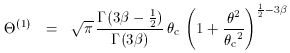(119)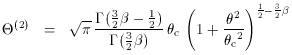(120)

which must then be convolved with the responses of the telescopes to calculate the structures that would be seen in practice. Values of0.7, and rc150 h100-1 kpc are typically obtained in fitting X-ray images of clusters to the structure defined by (113) and (119).

Values of the Hubble constant based on this distance estimation technique are now available for nine clusters. For the clusters with Sunyaev-Zel'dovich effects shown in Fig. 17, 19, 22 and 23, a detailed discussion of the fitting procedures used is given by Birkinshaw et al. (1991), Birkinshaw & Hughes (1994), Jones (1995), Holzapfel et al. (1997a), and Hughes & Birkinshaw (1998). The distances estimated for all nine clusters are displayed as luminosity distances as a function of redshift in Fig. 25. The error bars on the distance estimates are symmetrized errors taken from the individual papers and include systematic errors as well as random errors from uncertainties in the data.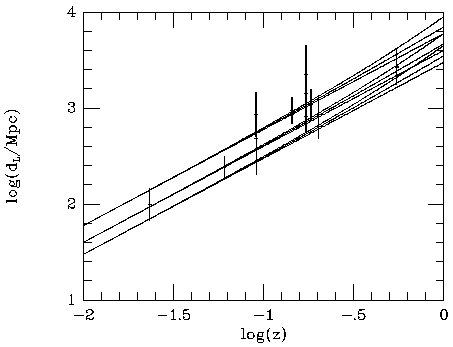Figure 25. A Hubble diagram based on the distances measured for the nine clusters Abell 1656, 2256, 478, 2142, 1413, 2163, 2218, and 665 and CL 0016+16 (Herbig et al. 1995; Myers et al. 1997; Grainge 1996; Holzapfel et al. 1997a; McHardy et al. 1990; Birkinshaw & Hughes 1994; Jones 1995; Birkinshaw et al. 1991; and Hughes & Birkinshaw 1996). Three values are shown for the distance of Abell 2218 (from Birkinshaw & Hughes, Jones, and McHardy et al.). The Hubble relation is drawn for H0 = 50, 75, and 100 km s-1 Mpc-1, with q0 = 0, 1/2, and 1. The current best fit is for a Hubble constant of about 60 km s-1 Mpc-1, with no strong constraint on q0, but no convincing error can be given because the distance estimates contain correlated systematic errors arising from the calibrations used (see text).

If the results in Fig. 25 are taken at face value, the measurements suggest a Hubble constant near 60 km s-1 Mpc-1, and have a scatter of about ± 20 km s-1 Mpc-1 (see the similar analysis of Furuzawa 1996). However, we cannot use this to conclude that H0 = 60 ± 10 km s-1 Mpc-1, as seems reasonable based on nine~measurements, since those measurements are not truly independent. In particular, only three different telescopes were used in the measurement of NSZ and only two in the measurement of NX, so that there are only about two independent X-ray calibrations and three independent Sunyaev-Zel'dovich effect calibrations in the set of results for H0. An improvement in the precision of the determination of H0, even in the absence of any other problems, must depend on convincing absolute calibrations of the Sunyaev-Zel'dovich effect and X-ray data.

There are a number of other systematic problems in using this technique. The most serious may be a selection effect, which causes the value of H0 to be biased low. If the model (118) for fn is modified to make the cluster atmosphere prolate or oblate, then the apparent X-ray and Sunyaev-Zel'dovich effect images of a cluster will be ellipsoidal, or circular if the symmetry axis lies along the line of sight. In the latter case it is clear that it will not be possible to tell that the cluster is aspherical based on the images: indeed, if the core radius of the gas distribution on the line of sight is larger by a factor Z than the core radii in the other two directions, then the density form factor becomes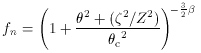(121)

and the expressions for(1) and(2) (119 and 120) remain valid, while the normalizations NX and NSZ both increase by a factor Z. The result is that a prolate gas distribution, with the symmetry axis along the line of sight, tends to give a higher central surface brightness than other gas distributions in which the same mass of gas is distributed spherically or with the symmetry axis perpendicular to the line of sight. This causes clusters elongated along the line of sight to be easier to detect in the X-ray or in the Sunyaev-Zel'dovich effect. Such clusters also give biased estimates of distance, since the true angular diameter distance is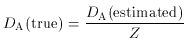(122)

if the distance is estimated using (117) not knowing that the cluster is elongated on the line of sight.

An indication of the importance of this effect is shown in Fig. 26, where the estimated value for the Hubble constant from CL 0016+16 is shown as a function of the intrinsic ellipticity (axial ratio) of an ellipsoidal model for the gas distribution. An ellipsoidal model is clearly preferred because of the non-circular X-ray and Sunyaev-Zel'dovich effect isophotes (Figs. 2, 23). The value of the Hubble constant derived by fitting the cluster by a spherical isothermal model is 68 km s-1 Mpc-1: it can be seen from the figure that by allowing ellipsoidal models with axial ratios as large as 2:1, values over the range 40-100 km s-1 Mpc-1 can be obtained.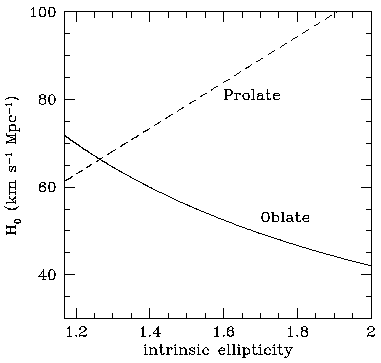Figure 26. The dependence of the Hughes & Birkinshaw's (1998) estimate of the value of the Hubble constant on assumptions about the oblateness or prolateness of CL 0016+16 in the extreme case where the cluster symmetry axis lies in the plane of the sky.

In order to avoid the selection bias in favor of clusters which are elongated along the line of sight, and hence of high surface brightness, and for which low estimates of the Hubble constant are produced, this technique must be applied to a sample of clusters selected without regard to their central surface brightness - perhaps clusters with total X-ray luminosities or flux densities above some limiting value. Such a selection is now possible using the high-sensitivity survey data recently returned by ROSAT (e.g., Ebeling et al. 1996). A corollary is that clusters which are intrinsically hard to study in the X-ray or the Sunyaev-Zel'dovich effect (and including Sunyaev-Zel'dovich effect non-detections) must be included in the set used to measure H0: the clusters with the weakest Sunyaev-Zel'dovich effects for their measured X-ray brightnesses are exactly those which imply larger values of H0 (albeit with larger observational errors). By contrast, the clusters in Fig. 25 were often selected based on having particularly strong Sunyaev-Zel'dovich effects, and are therefore likely to show an orientation bias. The size of this bias is not known at present, but is probably less than 30 per cent based on the distribution of X-ray axial ratios seen in other cluster samples.

In addition to this bias, there is a further contribution to the error in the estimated distance from the unknown intrinsic shape of cluster atmospheres. The range of observed shapes suggests an error of order 20 per cent is possible (Hughes & Birkinshaw 1998), and calculations of the evolution of cluster atmospheres confirm that this error estimate is reasonable (Roettiger et al. 1997; Yoshikawa et al. 1998).

A major component of the error in the estimates of the normalizations often arises from uncertainties in the parameters of the model (equation 121 or some more complicated function). This is particularly evident when the fits are based on older X-ray data (as, for example, Birkinshaw & Hughes 1994). The more recent X-ray imaging data from ROSAT substantially reduce the allowable range of parametersandc, so that this component of the error in the Hubble constant may be reduced. However, there is an intrinsic uncertainty in the types of gas model that are chosen to describe the atmosphere, and the extent to which they fail to represent aspects of the density and thermal structure of the gas that affect the distance estimate.

Modeling the gas appropriately is important because it is not the same gas that is responsible for the X-ray and Sunyaev-Zel'dovich effect signals that are used to determine the distance. The X-ray surface brightness is dominated by the densest parts of the cluster, since the X-ray emissivity of the gas is proportional to ne2 Te1/2, while the Sunyaev-Zel'dovich effect is dominated by the lower-density and hotter parts of the gas where the path lengths are longest. This effect is particularly important where single-dish measurements of the Sunyaev-Zel'dovich effect are used, while interferometer maps tend to resolve out structures on the largest angular scales. Uncertainties in the relationship between the contributions of low and high-density regions to the X-ray surface brightness and the Sunyaev-Zel'dovich effect can be avoided by making deep X-ray images, which trace the gas to sufficiently large radii that 90 or 95 per cent of the gas responsible for the Sunyaev-Zel'dovich effect is included. This means, however, tracing the cluster X-ray emission out to at least 10 core radii, at which the surface brightness has fallen to less than 10-3 of its central value, which often requires long integration times and careful treatment of the background in the X-ray detectors.

Thermal structure in the cluster atmosphere is harder to measure, and to achieve good accuracy in the distance estimates it is necessary to know about the temperature of the cluster gas out to 10 core radii. This is difficult, not only because of the low surface brightnesses of clusters at such radii, but also because of the lower angular resolution of X-ray detectors with useful spectral response. There is little clear information on the changes in temperature of cluster gas as a function of radius outside a few core radii, and an isothermal model (or sometimes a temperature model based on a bright nearby cluster, such as Coma; equation 73) are usually assumed. Systematic errors at the ten per cent level are likely from this uncertainty, and larger errors are possible for more extreme temperature profiles - hydrodynamical models of the evolution of cluster atmospheres (Roettiger et al. 1997; Yoshikawa et al. 1998) suggest that systematic errors of as much as 30 per cent and random errors of order 10 per cent in the Hubble constant may arise because of departures from isothermality.

A different type of density and temperature structure is often found in the central parts of clusters, where the high X-ray emissivity causes the cooling time of the gas to be short. The consequent decrease in central pressure causes a ``cooling flow'' to be established, with a slow inward drift of the atmosphere, an increase in the central X-ray surface brightness, and a decrease in the central gas temperature (e.g., Fabian et al. 1984). Since the central region in which there is a large change of gas properties is fairly small, it is still possible to use a model of the form (118) to describe the gas distribution, provided that the central X-ray brightness spike is excluded from the X-ray fit, and a corresponding change is made to the fitting for the Sunyaev-Zel'dovich effect. The Sunyaev-Zel'dovich effect will show less modification than the X-ray surface brightness in the presence of a cooling flow because the path length through the cooling region is relatively small, and there is only a small change of electron pressure in that region. However, the cooling gas may partly ``fill in'' the cm-wave microwave background diminution with free-free emission (Schlickeiser 1991), so that excluding the central region of a cluster from the fit may be important.

Even smaller-scale structure in cluster atmospheres can have an effect on the derived distance. If the intracluster gas is isothermal, but shows density clumping on a scale less than the resolution of the images, then the X-ray emissivity of a small element of gas is enhanced by a factor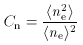(123)

while the value of < ne > is unchanged. Thus the cluster generates more X-ray emission than would be expected based on a uniform atmosphere, and hence the true angular diameter distance is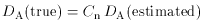(124)

so that with Cn > 1, the true value of the Hubble constant is smaller than the value estimated based on (117) without knowledge of the small-scale clumping.

Unlike the orientation bias, where averaging over a large number of clusters in random orientations with a known distribution of cluster shapes can correct the distance estimate, all cluster atmospheres are expected to be clumpy to some degree, and it is necessary to estimate the value of the clumping in the ``average'' cluster atmosphere, or to measure it in each cluster, in order to be sure that the distance estimate is not seriously in error. A theoretical estimate of the degree of clumping of the intracluster medium would be difficult, since it must take into account the processes that cause clumping (such as gas injection from the galaxies and energy input from galaxy motions) and that erase clumping (thermal conduction, gas-dynamical processes, and so on). If the clumping is strong and non-isothermal, then detailed X-ray spectroscopy may be able to measure the distribution of temperatures within a cluster, but it is unlikely that full account could be taken of a distribution of Cn (with an associated form factor, fC) over the cluster volume, nor that the full range of types of clumping could be tested in this way. At present it appears that the clumping of the intracluster medium is relatively weak, since if Cn is often large, then it would be expected to show significant variation from cluster to cluster, and the Hubble diagram (Fig. 25) would show stronger scatter than it does. However, the errors on the distance estimates in Fig. 25 at present cannot exclude values of Cn ~ 1.5, with consequent large systematic error in H0.

A variety of other potential problems with this method can be imagined. The Sunyaev-Zel'dovich effect signal could be contaminated by a background primordial anisotropy in the microwave background radiation (e.g., Cen 1998), or by the non-thermal Sunyaev-Zel'dovich effect of a cluster radio halo source, or by the kinematic Sunyaev-Zel'dovich effect, or by diffuse radio emission from cool gas (perhaps clumped into a population of spiral galaxies) towards the edge of the cluster. The X-ray signal could also be contaminated, perhaps by the inverse-Compton emission of relativistic electrons in the cluster radio halo source. Some of these effects are one-sided biases in the distance estimate, others would increase scatter in the Hubble diagram, but in general they should provide additional errors at the level of 10 per cent or less in the distance estimate (Birkinshaw et al. 1991; Holzapfel et al. 1997a).

The potential of this method for measuring the Hubble constant is only now starting to be realized, as better Sunyaev-Zel'dovich effect data become available. I expect a large increase in the number of clusters on a future Hubble diagram like Fig. 25, and that useful cosmological results will be obtained, especially as the maximum redshift at which an Sunyaev-Zel'dovich effect cluster is detected increases above 0.55. However, in view of the likely presence of residual systematic effects in the data and the low accuracy of any one measurement, I believe that it is premature to use them to estimate the values of the deceleration parameter and cosmological constant, as has been attempted recently by Kobayashi et al. (1996).